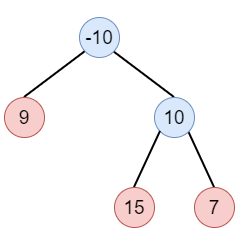# Binary Tree Postorder Traversal in Python Programming

Suppose we have a binary tree. We have to find the post order traversal of this tree using iterative approach. So if the tree is like −Then the output will be: [9,15,7,10,-10]

To solve this, we will follow these steps −

• if root is null, then return empty array

• create an array ret

• stack := define a stack with pair [root, 0]

• while stack is not empty −

• node := top of stack, then delete element from stack.

• if second value of node pair is 0, then

• current := first value of node pair

• insert a pair (current, 1) into stack

• if right of current is present

• insert a pair [right of current, 0] into stack

• if left of current is present −

• insert a pair [left of current, 0] into stack

• Otherwise when data of the first value node pair is not 0, then insert the data of the first value of node into res

• return res

Let us see the following implementation to get better understanding −

## Example

Live Demo

class TreeNode:
def __init__(self, data, left = None, right = None):
self.data = data
self.left = left
self.right = right
def insert(temp,data):
que = []
que.append(temp)
while (len(que)):
temp = que
que.pop(0)
if (not temp.left):
if data is not None:
temp.left = TreeNode(data)
else:
temp.left = TreeNode(0)
break
else:
que.append(temp.left)
if (not temp.right):
if data is not None:
temp.right = TreeNode(data)
else:
temp.right = TreeNode(0)
break
else:
que.append(temp.right)
def make_tree(elements):
Tree = TreeNode(elements)
for element in elements[1:]:
insert(Tree, element)
return Tree
class Solution(object):
def postorderTraversal(self, root):
if not root:
return []
res = []
stack = [[root,0]]
while stack:
node = stack[-1]
stack.pop()
if node== 0 :
current = node
stack.append([current,1])
if current.right:
stack.append([current.right,0])
if current.left:
stack.append([current.left,0])
else:
if node.data != 0:
res.append(node.data)
return res
ob = Solution()
root = make_tree([-10,9,10,None,None,15,7])
print(ob.postorderTraversal(root))

## Input

[-10,9,10,None,None,15,7]

## Output

[9, 15, 7, 10, -10]